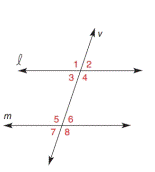Chapter 2.3, Problem 2E### Elementary Geometry for College St...

6th Edition
Daniel C. Alexander + 1 other
ISBN: 9781285195698

#### Solutions

Chapter
Section### Elementary Geometry for College St...

6th Edition
Daniel C. Alexander + 1 other
ISBN: 9781285195698
Textbook Problem
8 views

# In Exercises 1 to 6, l and m are cut by transversal v . On the basis of the information given, determine whether l must be parallel to m . m ∠ 2 = 65 °     and m ∠ 7 = 65 °To determine

To verify:

Whether 𝓁 must be parallel to m.

Explanation

Given:

𝓁 and m are cut by transversal v.

m2=65° and m7=65°.

Figure (1)

Approach:

(1) If two lines are cut by a transversal so that two corresponding angles are congruent, these lines are parallel.

(2) The vertically opposite angles are same in measure.

Calculation:

The given statement is, 𝓁 and m are cut by transversal v.

m2=65° and m7=65°

7 and 6 are vertically opposite angles

### Still sussing out bartleby?

Check out a sample textbook solution.

See a sample solution

#### The Solution to Your Study Problems

Bartleby provides explanations to thousands of textbook problems written by our experts, many with advanced degrees!

Get Started

#### Evaluate the expression sin Exercises 116. (2)3

Finite Mathematics and Applied Calculus (MindTap Course List)

#### True or False:

Study Guide for Stewart's Multivariable Calculus, 8th

#### The solution to xdydx2y=x3 is: a) y=ex3+Cx2 b) y=ex3+x2+C c) y=x3+x2+C d) y=x3+Cx2

Study Guide for Stewart's Single Variable Calculus: Early Transcendentals, 8th